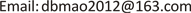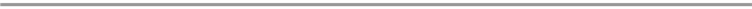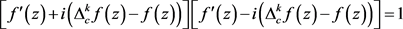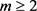﻿ 一类费马型微分–差分方程解的问题 Solution on Differential-Difference Equation of Fermat-Type

Pure Mathematics
Vol. 10  No. 03 ( 2020 ), Article ID: 34508 , 5 pages
10.12677/PM.2020.103023

Solution on Differential-Difference Equation of Fermat-Type

Bingmao Deng

School of Financial Mathematics and Statics, Guangdong University of Finance, Guangzhou GuangdongReceived: Feb. 17th, 2020; accepted: Mar. 4th, 2020; published: Mar. 13th, 2020ABSTRACT

In this paper, we mainly discussed entire solutions with finite order of the following Fermat type differential-difference equation ${\left[{f}^{\prime }\left(z\right)\right]}^{2}+{\left[{\Delta }_{c}^{k}f\left(z\right)-f\left(z\right)\right]}^{2}=1$ and obtained some interesting results.

Keywords:Fermat-Type Equation, Entire Function, Differential-Difference Equation1. 引言及主要结果,

$\underset{j=0}{\overset{k}{\sum }}{b}_{j}{a}^{j}=\frac{1}{i}$,$\underset{j=0}{\overset{k}{\sum }}{b}_{j}{\left(-a\right)}^{j}=-\frac{1}{i}$.

2012年，Liu等人  研究了以下费马型差分–微分方程，并获得了以下结论。

${\left[{f}^{\prime }\left(z\right)\right]}^{2}+{\left[f\left(z+c\right)-f\left(z\right)\right]}^{2}=1$. (1.1)

$f\left(z\right)=\mathrm{sin}\left(2z+Bi\right)/2$,

${\left[{f}^{\prime }\left(z\right)\right]}^{2}+{\left[{\Delta }_{c}^{k}f\left(z\right)-f\left(z\right)\right]}^{2}=1$. (1.2)

$f\left(z\right)\equiv ±1$，或者，其中 $a,b,d$ 是常数，并且满足 ${\text{e}}^{kac}={\left(-1\right)}^{k}$

${\left({\text{e}}^{ac}-1\right)}^{k}\ne 1$,$a=i{\left({\text{e}}^{ac}-1\right)}^{k}-i$.

$f\left(z\right)\equiv ±1$，或者

$f\left(z\right)=\frac{1}{3}\mathrm{sin}\left(3z+bi\right)+d$，其中 $b,d$ 是常数， $c=\left(\pi +n\pi \right)/3$

2. 一些引理

$\underset{i=1}{\overset{n}{\sum }}{f}_{i}\left(z\right){\text{e}}^{{g}_{i}\left(z\right)}\equiv 0$ ;

$T\left(r,{f}_{j}\right)=S\left(r,{\text{e}}^{{g}_{h}-{g}_{l}}\right)$,$r\to \infty$,$r\notin E$,

${f}_{i}\left(z\right)\equiv 0\left(1\le i\le n\right)$

$f\left(z\right)={z}^{k}P\left(z\right){\text{e}}^{Q\left(z\right)}$,

3. 定理3的证明

$f\left(z\right)$ 是方程(1.2)的有穷级整函数解，将(1.3)改写成下式。 (3.1)(3.2)

${f}^{\prime }\left(z\right)=\frac{{\text{e}}^{p\left(z\right)}+{\text{e}}^{-p\left(z\right)}}{2}$, (3.3)

${\Delta }_{c}^{k}f\left(z\right)-f\left(z\right)=\frac{{\text{e}}^{p\left(z\right)}-{\text{e}}^{-p\left(z\right)}}{2i}$. (3.4)

${\left[{\Delta }_{c}^{k}f\left(z\right)-f\left(z\right)\right]}^{\prime }=\underset{j=0}{\overset{k}{\sum }}{\left(-1\right)}^{k-j}{C}_{k}^{j}{f}^{\prime }\left(z+jc\right)-{f}^{\prime }\left(z\right)=\frac{{p}^{\prime }\left(z\right)}{2i}\left[{\text{e}}^{p\left(z\right)}+{\text{e}}^{-p\left(z\right)}\right]$. (3.5)

${f}^{\prime }\left(z+jc\right)=\frac{1}{2}\left[{\text{e}}^{p\left(z+jc\right)}+{\text{e}}^{-p\left(z+jc\right)}\right]$. (3.6)

$\underset{j=0}{\overset{k}{\sum }}{\left(-1\right)}^{k-j}{C}_{k}^{j}\left[{\text{e}}^{p\left(z+jc\right)}+{\text{e}}^{-p\left(z+jc\right)}\right]-\left[{\text{e}}^{p\left(z\right)}+{\text{e}}^{-p\left(z\right)}\right]=-i{p}^{\prime }\left(z\right)\left[{\text{e}}^{p\left(z\right)}+{\text{e}}^{-p\left(z\right)}\right]$. (3.7)

$\left[i{p}^{\prime }\left(z\right)-1+{\left(-1\right)}^{k}\right]\left[{\text{e}}^{p\left(z\right)}+{\text{e}}^{-p\left(z\right)}\right]+\underset{j=1}{\overset{k}{\sum }}{\left(-1\right)}^{k-j}{C}_{k}^{j}\left[{\text{e}}^{p\left(z+jc\right)}+{\text{e}}^{-p\left(z+jc\right)}\right]=0$. (3.8)，则对任意的 $0\le j，均有

$\rho \left({\text{e}}^{p\left(z+jc\right)-p\left(z+lc\right)}\right)=m-1\ge 1$, 且 $\rho \left({\text{e}}^{p\left(z+jc\right)+p\left(z+lc\right)}\right)=m\ge 2$.

(3.8)式两边同时乘以 ${\text{e}}^{p\left(z\right)}$，并化简，可得

$\left[ia-1+{\left(-1\right)}^{k}\right]\left[{\text{e}}^{2p\left(z\right)}+1\right]+\underset{j=1}{\overset{k}{\sum }}{\left(-1\right)}^{k-j}{C}_{k}^{j}\left[{\text{e}}^{p\left(z\right)+p\left(z+jc\right)}+{\text{e}}^{p\left(z\right)-p\left(z+jc\right)}\right]=0$.

$\left[ia-1+{\left(-1\right)}^{k}\right]\left[{\text{e}}^{2p\left(z\right)}+1\right]+\underset{j=1}{\overset{k}{\sum }}{\left(-1\right)}^{k-j}{C}_{k}^{j}\left[{\text{e}}^{2p\left(z\right)+ajc}+{\text{e}}^{-ajc}\right]=0$. (3.9)

${\text{e}}^{2p\left(z\right)}\left[\underset{j=0}{\overset{k}{\sum }}{\left(-1\right)}^{k-j}{C}_{k}^{j}{\text{e}}^{ajc}+ia-1\right]+\left[\underset{j=0}{\overset{k}{\sum }}{\left(-1\right)}^{k-j}{C}_{k}^{j}{\text{e}}^{-ajc}+ia-1\right]=0$. (3.10)

$\left\{\begin{array}{l}\underset{j=0}{\overset{k}{\sum }}{\left(-1\right)}^{k-j}{C}_{k}^{j}{\text{e}}^{ajc}+ia-1=0;\\ \underset{j=0}{\overset{k}{\sum }}{\left(-1\right)}^{k-j}{C}_{k}^{j}{\text{e}}^{-ajc}+ia-1=0.\end{array}$

${\text{e}}^{2p\left(z\right)}=-\frac{\underset{j=0}{\overset{k}{\sum }}{\left(-1\right)}^{k-j}{C}_{k}^{j}{\text{e}}^{-ajc}+ia-1}{\underset{j=0}{\overset{k}{\sum }}{\left(-1\right)}^{k-j}{C}_{k}^{j}{\text{e}}^{ajc}+ia-1}$,

$\left\{\begin{array}{l}{\left({\text{e}}^{ac}-1\right)}^{k}+ia-1=0;\\ {\left({\text{e}}^{-ac}-1\right)}^{k}+ia-1=0.\end{array}$ (3.11)

$f\left(z\right)=\frac{{\text{e}}^{az+b}-{\text{e}}^{-az-b}}{2a}+d=\frac{1}{ai}\mathrm{sin}\left[aiz+bi\right]+d$.

Solution on Differential-Difference Equation of Fermat-Type[J]. 理论数学, 2020, 10(03): 162-166. https://doi.org/10.12677/PM.2020.103023

1. 1. Hayman, W.K. (1964) Meromorphic Functions. Clarendon Press, Oxford.

2. 2. Yang, L. (1993) Value Distribution Theory. Springer-Verlag, Berlin.

3. 3. Yi, H.X. and Yang, C.C. (2003) Uniqueness Theory of Meromorphic Functions. Science Press, Beijing.

4. 4. Gross, F. (1966) On the Equation fn + gn = 1. Bulletin of the American Mathematical Society, 72, 86-88. https://doi.org/10.1090/S0002-9904-1966-11429-5

5. 5. Gross, F. (1966) On the Equation fn + gn = hn. The American Mathematical Monthly, 73, 1093-1096. https://doi.org/10.2307/2314644

6. 6. Yang, C.C. and Li, P. (2004) On the Transcendental Solutions of a Certain Type of Nonlinear Differential Equations. Archiv der Mathematik, 82, 442-448. https://doi.org/10.1007/s00013-003-4796-8

7. 7. Chen, M.F., Gao, Z.S. and Du, Y.F. (2017) Existence of Entire Solutions of Some Non-Linear Differential-Difference Equations. Journal of Inequalities and Applications, 90, 17 p. https://doi.org/10.1186/s13660-017-1368-1

8. 8. Chiang, Y.M. and Feng, S.J. (2008) On the Nevanlinna Characteristic of f(z+η) and Difference Equations in the Complex Plane. The Ramanujan Journal, 16, 105-129. https://doi.org/10.1007/s11139-007-9101-1

9. 9. Laine, I., Rieppo, J. and Silvennoinen, H. (2005) Remarks on Complex Difference Equations. Computational Methods and Function Theory, 5, 77-88. https://doi.org/10.1007/BF03321087

10. 10. Halburd, R.G. and Korhonen, R.J. (2006) Difference Analogue of the Lemma on the Logarithmic Derivative with Applications to Difference Equations. Journal of Mathematical Analysis and Applications, 314, 477-487. https://doi.org/10.1016/j.jmaa.2005.04.010

11. 11. Liu, K., Cao, T.B. and Cao, H.Z. (2012) Entire Solutions of Fermat Type Differential-Difference Equations. Archiv der Mathematik, 99, 147-155. https://doi.org/10.1007/s00013-012-0408-9

12. 12. Liu, K. (2015) Fermat Type Differential and Difference Equations. Electronic Journal of Differential Equations, 159, 1-10. https://doi.org/10.1186/s13662-015-0534-3

13. 13. Gao, L.Y. (2017) On Entire Solutions of Two Types of Systems of Complex Differential-Difference Equation. Acta Mathematica Scientia, 37B, 187-194. https://doi.org/10.1016/S0252-9602(16)30124-2

14. 14. 高凌云. 关于一类复微分-差分方程组的解[J]. 数学年刊, 2017, 38A(1): 23-30.

15. 15. Gao, L.Y. (2016) Entire Solutions of Two Types of Systems of Complex Differential-Difference Equations. Acta Mathematica Scientia, 59, 677-684.